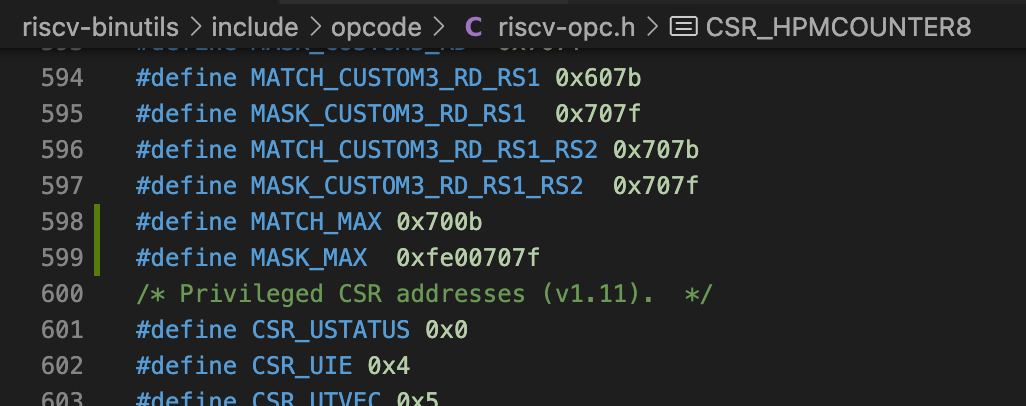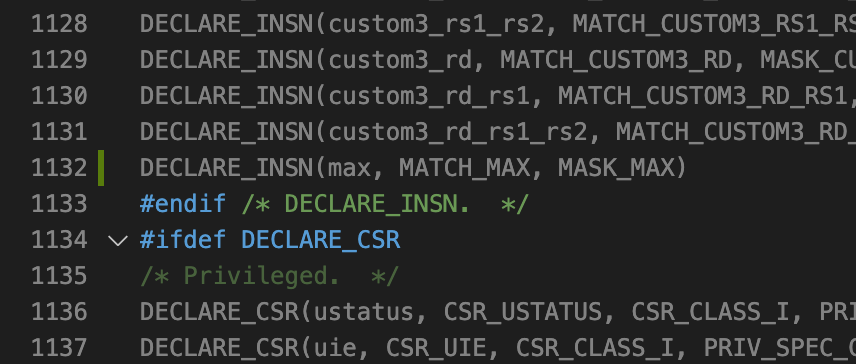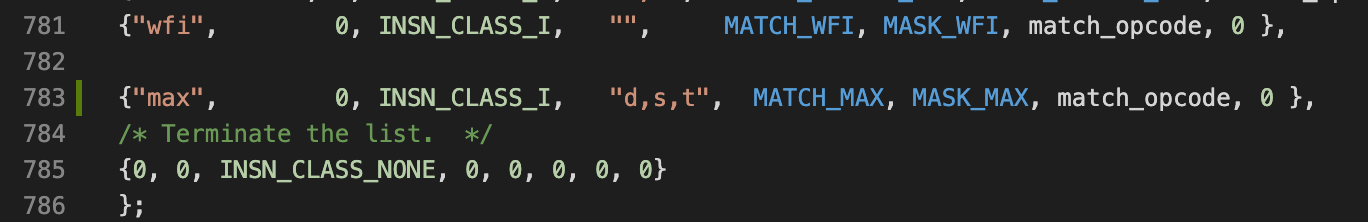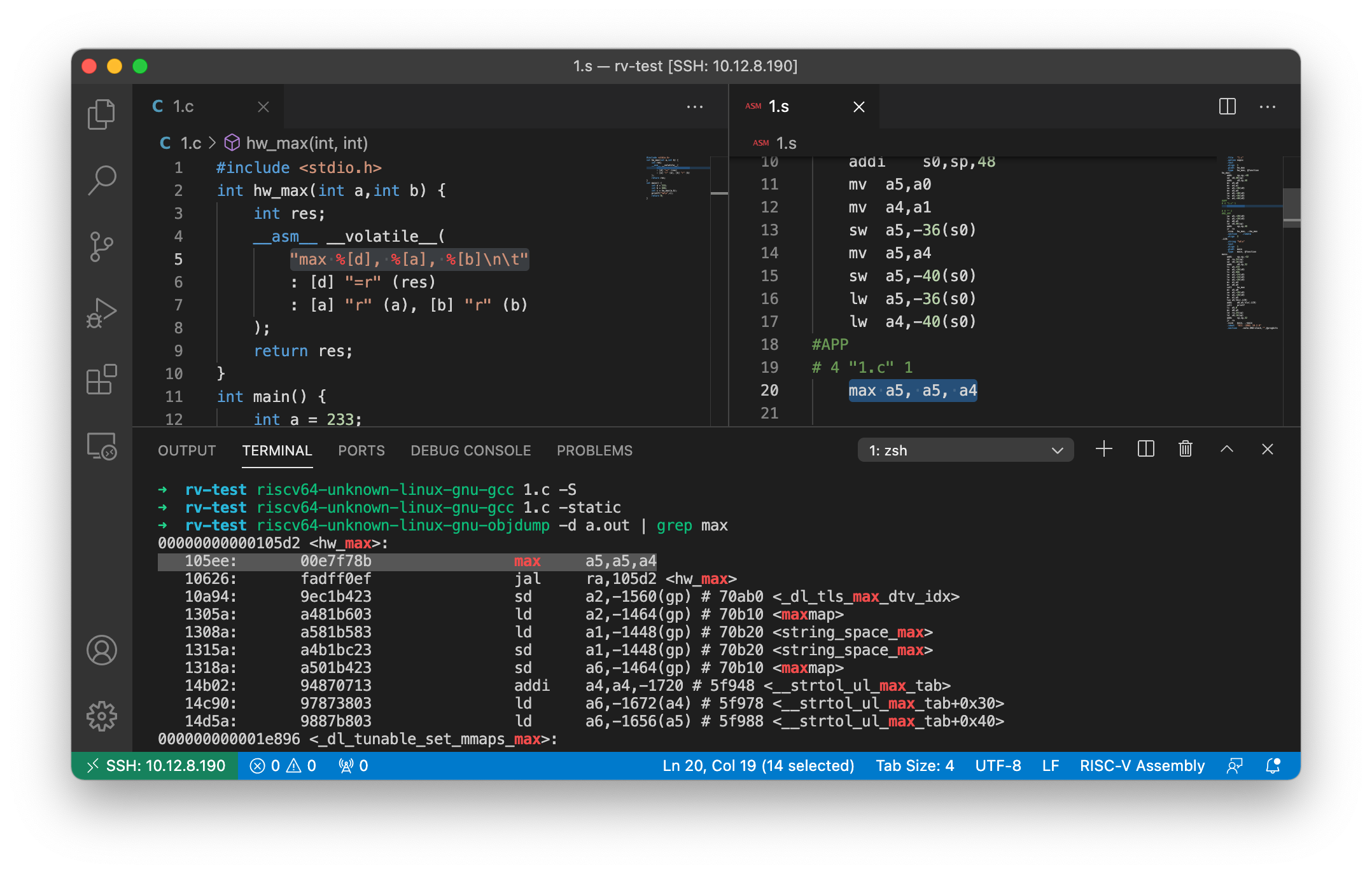# 修改binutils在RISC-V上添加汇编指令

## 2. 准备环境

sudo apt-get install autoconf automake autotools-dev curl python3 libmpc-dev libmpfr-dev libgmp-dev gawk build-essential bison flex texinfo gperf libtool patchutils bc zlib1g-dev libexpat-dev
sudo mkdir /opt/riscv
sudo chown $USER:$USER /opt/riscv # $USER是自己的用户，这样后续操作可以省去需要root权限的麻烦 git clone git@github.com:riscv/riscv-gnu-toolchain.git cd riscv-gnu-toolchain ./configure --prefix=/opt/riscv make linux -j 16 # 16根据自己的CPU线程数修改 在安装完成后，最好将/opt/riscv/bin添加到$PATH环境变量中，以便直接使用。

echo "export PATH=/opt/riscv/bin:\$PATH" >> ~/.zshrc # 如果你使用的是zsh，如果是默认的bash改成.bashrc即可

## 3. 使用riscv-opcodes生成编译器所需宏定义

git clone git@github.com:riscv/riscv-opcodes.git
cd riscv-opcodes
code opcodes-custom # code指vscode，可以换成你喜欢的编辑器

@custom0            rd rs1 imm12 14..12=0 6..2=0x02 1..0=3
@custom0.rs1        rd rs1 imm12 14..12=2 6..2=0x02 1..0=3
@custom0.rs1.rs2    rd rs1 imm12 14..12=3 6..2=0x02 1..0=3
@custom0.rd         rd rs1 imm12 14..12=4 6..2=0x02 1..0=3
@custom0.rd.rs1     rd rs1 imm12 14..12=6 6..2=0x02 1..0=3
@custom0.rd.rs1.rs2 rd rs1 imm12 14..12=7 6..2=0x02 1..0=3

max                 rd rs1 rs2 31..25=0 14..12=7 6..2=0x02 1..0=3

python3 parse_opcodes -c < opcodes-custom > def.h

#define MATCH_MAX 0x700b
// ...
DECLARE_INSN(max, MATCH_MAX, MASK_MAX)

## 4. 修改编译器的binutilsconst struct riscv_opcode riscv_opcodes[] =
{
/* name,     xlen, isa,   operands, match, mask, match_func, pinfo.  */

{"max",        0, INSN_CLASS_I,   "d,s,t",  MATCH_MAX, MASK_MAX, match_opcode, 0 },make clean
make linux -j 16

## 5. 编写程序测试：

#include <stdio.h>
int hw_max(int a,int b) {
int res;
__asm__ __volatile__(
"max %[d], %[a], %[b]\n\t"
: [d] "=r" (res)
: [a] "r" (a), [b] "r" (b)
);
return res;
}
int main() {
int a = 233;
int b = 666;
int c = hw_max(a,b);
printf("%d\n",c);
return 0;
}## 6. 参考资料

Adding custom instruction to RISCV ISA and running it on gem5 and spike

Syntacore RISCV 工具链使用（五）gcc工具链的生成与添加自定义指令集